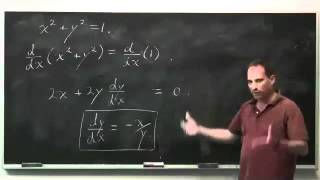﻿ AP Calculus Videos

# AP Calculus

• ### Worldwide Calculus: Average Rates of Change

Lecture on 'Average Rates of Change' from 'Worldwide Differential Calculus' and 'Worldwide AP Calculus'. For more lecture videos and \$10 digital textbooks, visit www.centerofmath.org

• ### Worldwide Calculus: Prelude to Instantaneous Rates of Change

Lecture on 'Prelude to Instantaneous Rates of Change' from 'Worldwide Differential Calculus' and 'Worldwide AP Calculus'. For more lecture videos and \$10 digital textbooks, visit www.centerofmath.org.

• ### Worldwide Calculus: Limits and Continuity

Lecture on 'Limits and Continuity' from 'Worldwide Differential Calculus' and 'Worldwide AP Calculus'. For more lecture videos and \$10 digital textbooks, visit www.centerofmath.org

• ### Worldwide Calculus: Instantaneous Rates of Change: The Derivative

Lecture on 'Instantaneous Rates of Change: The Derivative' from 'Worldwide Differential Calculus' and 'Worldwide AP Calculus'. For more lecture videos and \$10 digital textbooks, visit www.centerofmath.org.

• ### Worldwide Calculus: Extrema and the Mean Value Theorem

Lecture on 'Extrema and the Mean Value Theorem' from 'Worldwide Differential Calculus' and 'Worldwide AP Calculus'. For more lecture videos and \$10 digital textbooks, visit www.centerofmath.org.

• ### Worldwide Calculus: Higher-Order Derivatives

Lecture on 'Higher-Order Derivatives' from 'Worldwide Differential Calculus' and 'Worldwide AP Calculus'. For more lecture videos and \$10 digital textbooks, visit www.centerofmath.org.

• ### Worldwide Calculus: The Power Rule for Integer Powers

Lecture on 'The Power Rule for Integer Power's from 'Worldwide Differential Calculus' and 'Worldwide AP Calculus'. For more lecture videos and \$10 digital textbooks, visit www.centerofmath.org.

• ### Worldwide Calculus: The Product and Quotient Rules

Lecture on 'The Product and Quotient Rules' from 'Worldwide Differential Calculus' and 'Worldwide AP Calculus'. For more lecture videos and \$10 digital textbooks, visit www.centerofmath.org.

• ### Worldwide Calculus: The Chain Rule

Lecture on 'The Chain Rule' from 'Worldwide Differential Calculus' and 'Worldwide AP Calculus'. For more lecture videos and \$10 digital textbooks, visit www.centerofmath.org.

• ### Worldwide Calculus: The Exponential Function

Lecture on 'The Exponential Function' from 'Worldwide Differential Calculus' and 'Worldwide AP Calculus'. For more lecture videos and \$10 digital textbooks, visit www.centerofmath.org.

• ### Worldwide Calculus: The Natural Logarithm

Lecture on 'The Natural Logarithm' from 'Worldwide Differential Calculus' and 'Worldwide AP Calculus'. For more lecture videos and \$10 digital textbooks, visit www.centerofmath.org.

• ### Worldwide Calculus: General Exponential and Logarithmic Functions

Lecture on 'General Exponential and Logarithmic Functions' from 'Worldwide Differential Calculus' and 'Worldwide AP Calculus'. For more lecture videos and \$10 digital textbooks, visit www.centerofmath.org.

• ### Worldwide Calculus: Trigonometric Functions: Sine and Cosine

Lecture on 'Trigonometric Functions: Sine and Cosine' from 'Worldwide Differential Calculus' and 'Worldwide AP Calculus'. For more lecture videos and \$10 digital textbooks, visit www.centerofmath.org.

• ### Worldwide Calculus: The Other Trigonometric Functions

Lecture on 'The Other Trigonometric Functions' from 'Worldwide Differential Calculus' and 'Worldwide AP Calculus'. For more lecture videos and \$10 digital textbooks, visit www.centerofmath.org.

• ### Worldwide Calculus: Inverse Trig Functions

Lecture on 'Inverse Trig Functions' from 'Worldwide Differential Calculus' and 'Worldwide AP Calculus'. For more lecture videos and \$10 digital textbooks, visit www.centerofmath.org.

• 36:31 Popular### Worldwide Calculus: Implicitly Deﬁned Functions

Lecture on 'Implicitly Defined Functions' from 'Worldwide Differential Calculus' and 'Worldwide AP Calculus'. For more lecture videos and \$10 digital textbooks, visit www.centerofmath.org.

• ### Worldwide Calculus: Related Rates

Lecture on 'Related Rates' from 'Worldwide Differential Calculus' and 'Worldwide AP Calculus'. For more lecture videos and \$10 digital textbooks, visit www.centerofmath.org.

• ### Worldwide Calculus: Graphing

Lecture on 'Graphing' from 'Worldwide Differential Calculus' and 'Worldwide AP Calculus'. For more lecture videos and \$10 digital textbooks, visit www.centerofmath.org.

• ### Worldwide Calculus: Optimization

Lecture on Optimization from 'Worldwide Differential Calculus' and 'Worldwide AP Calculus'. For more lecture videos and \$10 digital textbooks, visit www.centerofmath.org.

• ### Worldwide Calculus: Linear Approximation, Differentials, and Newton's Method

Lecture on 'Linear Approximation, Differentials, and Newton's Method' from 'Worldwide Differential Calculus' and 'Worldwide AP Calculus'. For more lecture videos and \$10 digital textbooks, visit www.centerofmath.org.

• ### Worldwide Calculus: Indeterminate Forms and L´Hôpital's Rule

Lecture on 'Indeterminate Forms and L´Hôpital's Rule' from 'Worldwide Differential Calculus' and 'Worldwide AP Calculus'. For more lecture videos and \$10 digital textbooks, visit www.centerofmath.org.

• ### Worldwide Calculus: Anti-Derivatives

Lecture on 'Anti-Derivatives' from 'Worldwide Differential Calculus', 'Worldwide Integral calculus', and 'Worldwide AP Calculus'. For more lecture videos and \$10 digital textbooks, visit www.centerofmath.org.